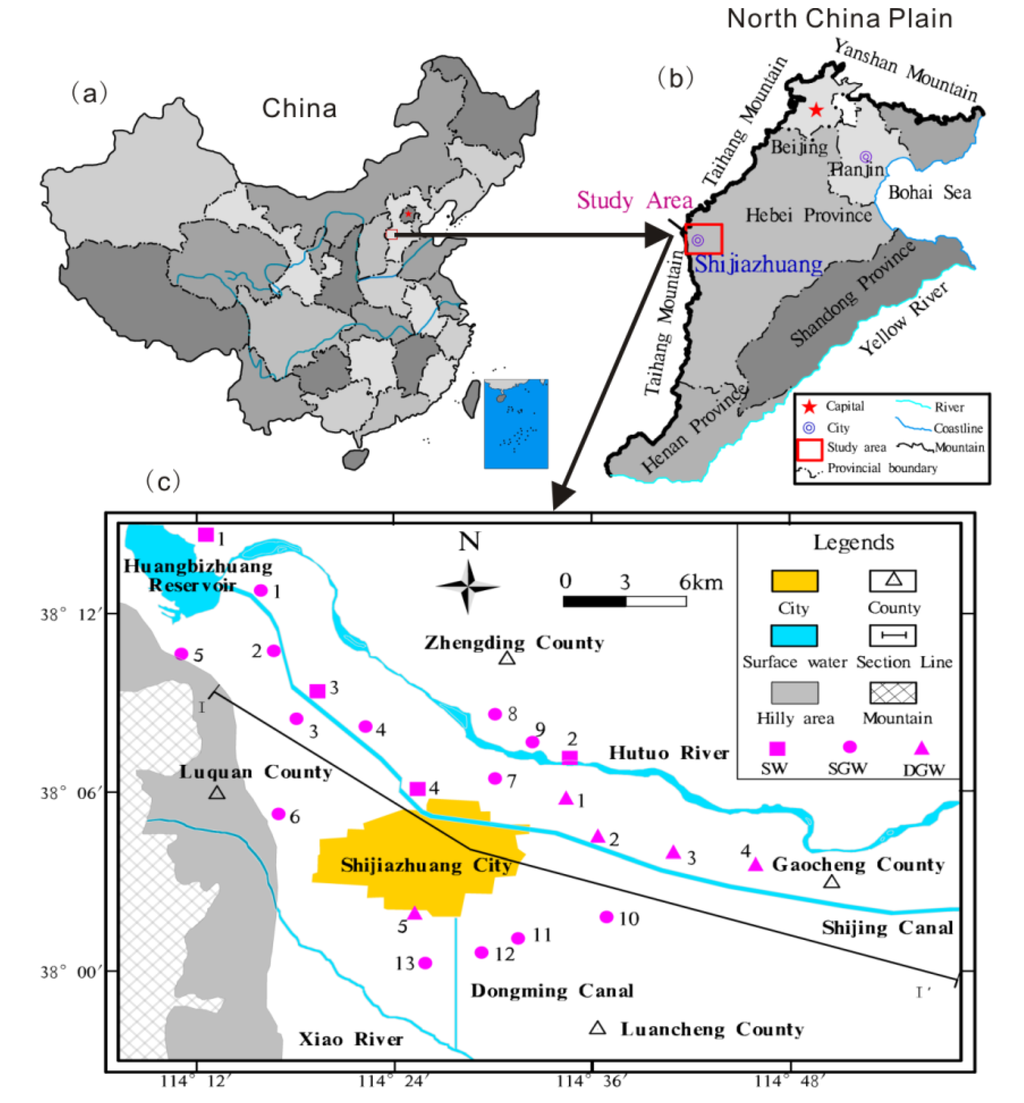Open AccessArticle

# Evaluating the Sources and Fate of Nitrate in the Alluvial Aquifers in the Shijiazhuang Rural and Suburban Area, China: Hydrochemical and Multi-Isotopic Approaches

by 1,2, 1,2, 1,2,*, 1,2 and 1,2
1
School of Environmental Studies, China University of Geosciences, Wuhan 430074, China
2
State Key Laboratory of Biogeology and Environmental Geology (China University of Geosciences), Wuhan 430074, China
*
Author to whom correspondence should be addressed.
Academic Editor: Jun Xu
Water 2015, 7(4), 1515-1537; https://doi.org/10.3390/w7041515
Received: 7 December 2014 / Revised: 26 March 2015 / Accepted: 27 March 2015 / Published: 9 April 2015
The identification of the sources and fate of NO$$_{3}^{-}$$ contaminants is important to protect the water quality of aquifer systems. In this study, NO$$_{3}^{-}$$ contaminated groundwater from the drinking water wells in the Shijiazhuang area, China, was chemically (NO$$_{3}^{-}$$/Cl$$^{\rm{{-}}}$$ ratio) and isotopically ($$δ$$$$^{\rm{15}}$$N$$_{\rm{NO3}}$$, $$\delta$$$$^{\rm{18}}$$O$$_{\rm{NO3}}$$ and $$\delta$$$$^{\rm{13}}$$C$$_{\rm{DOC}}$$; $$\delta$$$$^{\rm{2}}$$H$$_{\rm{H2O}}$$, $$\delta$$$$^{\rm{18}}$$O$$_{\rm{H2O}}$$) characterized to identify the sources of NO$$_{3}^{-}$$ and address subsequent biogeochemical processes. The positive correlations between dominant anions and cations suggested that the dissolution of calcium carbonate and gypsum minerals was the most effective process in the groundwater. Elevated concentrations of NO$$_{3}^{-}$$, Cl$$^{\rm{{-}}}$$ and Mg$$^{\rm{2+}}$$ could be related to the wastewater irrigation and usage of fertilizers. The natural water in the study area originated primarily from precipitation and experienced a limited extent of evaporation, as demonstrated by measurements of $$\delta$$$$^{\rm{2}}$$H$$_{\rm{H2O}}$$ and $$\delta$$$$^{\rm{18}}$$O$$_{\rm{H2O}}$$. A cross-plot of $$\delta$$$$^{\rm{15}}$$N$$_{\rm{NO3}}$$ vs. $$\delta$$$$^{\rm{18}}$$O$$_{\rm{NO3}}$$ gave an enrichment of the 15N isotope relative to the 18O isotope by a factor of 2. A further insight into the denitrification process was obtained by the synergistic changes in $$\delta$$$$^{\rm{13}}$$C$$_{\rm{DOC}}$$ and $$\delta$$$$^{\rm{15}}$$N$$_{\rm{NO3}}$$ values, confirming that a low extent of denitrification occurred. Nitrification processes were evaluated by means of $$\delta$$$$^{\rm{18}}$$O$$_{\rm{NO3}}$$ and $$\delta$$$$^{\rm{18}}$$O$$_{\rm{H2O}}$$. The initial $$\delta$$$$^{\rm{15}}$$N$$_{\rm{NO3}}$$ value(s) of the NO$$_{3}^{-}$$ source(s) were roughly estimated between 2‰ and 5‰. Based on the level of natural NO$$_{3}^{-}$$, anthropogenic activities were considered the main reason for the elevated NO$$_{3}^{-}$$ concentration of the shallow groundwater. NH$$_{4}^{+}$$ fertilizers were the major source of NO$$_{3}^{-}$$ in the non-wastewater irrigated area, while wastewater was regarded as the primary source of NO$$_{3}^{-}$$ in the wastewater-irrigated area. A low content of NO$$_{3}^{-}$$ in deep groundwater might mainly be influenced by precipitation and soil organic N that was involved in denitrification reactions. Some of the deep groundwater samples could have been contaminated by wastewater. The mixing process of multiple NO$$_{3}^{-}$$ sources was identified as another important factor affecting the NO$$_{3}^{-}$$ concentration of the groundwater in the study area. The combined use of $$\delta$$$$^{\rm{15}}$$N$$_{\rm{NO3}}$$, $$\delta$$$$^{\rm{18}}$$O$$_{\rm{NO3}}$$ and $$\delta$$$$^{\rm{13}}$$C$$_{\rm{DOC}}$$ results and hydrochemical data (NO$$_{3}^{-}$$/Cl$$^{\rm{{-}}}$$ ratios) gives an insight into the mixing effect of different NO$$_{3}^{-}$$ sources and processes affecting NO$$_{3}^{-}$$ concentration under conditions of intensive land-use activities. View Full-Text
Keywords:
Show FiguresFigure 1

MDPI and ACS Style

Zhang, Y.; Zhou, A.; Zhou, J.; Liu, C.; Cai, H.; Liu, Y.; Xu, W. Evaluating the Sources and Fate of Nitrate in the Alluvial Aquifers in the Shijiazhuang Rural and Suburban Area, China: Hydrochemical and Multi-Isotopic Approaches. Water 2015, 7, 1515-1537.

Show more citation formats Show less citations formats

### Article Access Map

1
Only visits after 24 November 2015 are recorded.